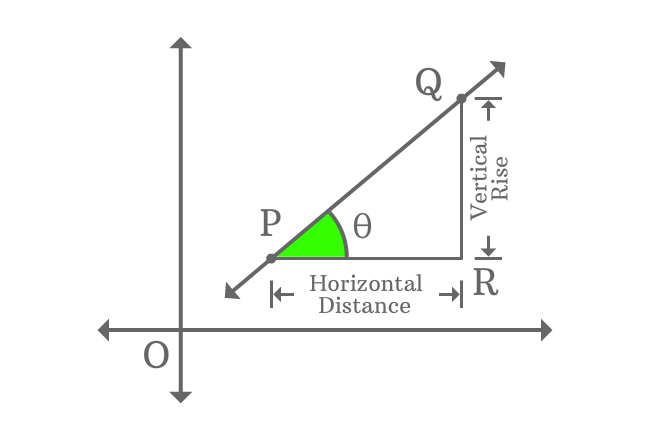# Slope of a Straight Line in trigonometric form

## Formula

$m \,=\, \tan{\theta}$

The tangent of inclination of a straight line is called the slope of straight line.

### Introduction

$\overleftrightarrow{PQ}$ is a straight line in Cartesian coordinate system with some inclination.Draw a parallel line to $x$-axis from point $P$ and also draw a perpendicular line towards $x$-axis from point $Q$. The both lines are intersected at point $R$ perpendicularly and it formed a right triangle ($\Delta RPQ$) geometrically.

The lengths of $\overline{QR}$ and $\overline{PR}$ represent vertical rise and horizontal distance of points of $P$ and $Q$ of the straight line. The slope of this straight line is ratio of vertical rise to horizontal distance of points of the straight line.

The slope of straight line $\overleftrightarrow{PQ}$ is represented by letter $m$ mathematically in geometric system.

$m \,=\, \dfrac{Vertical \, Rise}{Horizontal \, Distance}$

$\implies m \,=\, \dfrac{QR}{PR}$

$\Delta RPQ$ is a right triangle and, $\overline{QR}$ and $\overline{PR}$ are opposite side and adjacent side of the right triangle. Theta ($(\theta)$) is inclination of the straight line and it is also angle of the right triangle. The ratio of $QR$ to $PR$ is tan of angle theta as per trigonometry.

$\,\,\, \therefore \,\,\,\,\,\, m \,=\, \tan{\theta}$

The derivation has proved that the slope of a straight line is equal to tangent of the inclination of the straight line.

Latest Math Topics
Jun 26, 2023
Jun 23, 2023

Latest Math Problems
Jul 01, 2023
Jun 25, 2023
###### Math Questions

The math problems with solutions to learn how to solve a problem.

Learn solutions

Practice now

###### Math Videos

The math videos tutorials with visual graphics to learn every concept.

Watch now

###### Subscribe us

Get the latest math updates from the Math Doubts by subscribing us.